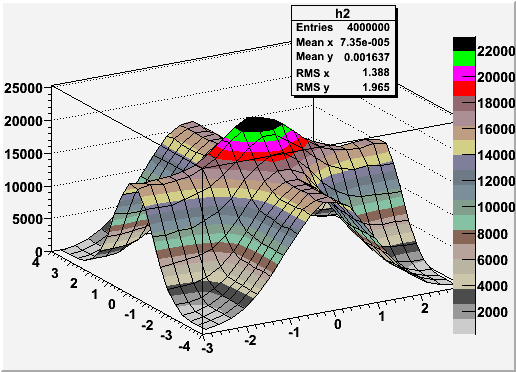# Invert 2D Histogram

Dear List,

I want to invert a 2D histogram by normalising each bin content to the maximum value across the distribution, and then subracting from 1. The histogram itself was generated using data from a function defined in a TF2 constructor.

I’ve extracted the maximum value across the bins, but I’m having some trouble normalising and inverting. Is there an elegant solution for this?

See sample script and plot below.

Many thanks in advance!

``````#include "TMath.h"
#include "TF1.h"
#include "TF2.h"
gROOT->Reset();
gStyle->SetOptStat(0);
gStyle->SetPalette(1);
//
void DQANew1()
{
//Define some sample 2D gaussian function
//
TCanvas *c1 = new TCanvas("c1","the fit canvas",500,400);
TF2 *f2 = new TF2("f2","sqrt((exp(-0.5*((x-)^2/(^2))))^2/^2+(exp(-0.5*((y-)^2/(^2))))^2/^2)",-3,3,-4,4);
Double_t params[] = {0,0.5,1,0.5,1.5,1};
//
//Set Paramaters
f2->SetParameters(params);
//
//Test 2D function
f2->Draw();
//
//:::::::::::Now plot function as 2D histogram
TCanvas *c2 = new TCanvas("c2","the fit canvas",500,400);
TH2F *h2 = new TH2F("h2","",20,-3.,3.,20,-4.,4.);
h2->SetFillColor(46);
h2->FillRandom("f2",4000000);
h2->Draw("surf1z");
//
// Extract maxmimum value
printf("Get Maximum bin value = %g\n",h2->GetMaximum());
Double_t MaxBinVal = h2->GetMaximum();
//
//check that MaxBinVal has been captured
printf("MaxBinVal = %g\n",h2->GetMaximum());
//
//::::::::::Now normalise and subtract from 1 to invert h2
TCanvas *c3 = new TCanvas("c3","the fit canvas",500,400);
TF2 *f3 = new TF2("f3","1-((sqrt((exp(-0.5*((x-)^2/(^2))))^2/^2+(exp(-0.5*((y-)^2/(^2))))^2/^2))/MaxBinVal)",-3,3,-4,4);
Double_t params1[] = {0,0.5,1,0.5,1.5,1};
f3->SetParameters(params1);
TH2F *h3 = new TH2F("h3","",20,-3.,3.,20,-4.,4.);
h3->FillRandom("f3",4000000);
h3->Draw("surf1z");
}``````

Plot that I would like to invertHave you tried to use the histogram operations Add, Divide etc … ?

root.cern.ch/doc/master/classTH2F.html

yes, using [1/(h2->GetMaximum())] will normalise the histogram so that the z -axis is scaled to 1. But using 1-(1/(h2->GetMaximum())) to invert doesn’t do anything! It seems that scaling only changes the appearance of the visual display but not the actual histogram bin contents?

Subtracting the normalised 2D histogramed from 1 doesn’t work either…I keep gettig operator or interpreter errors

No, it changes the histogram …

Can you post an example showing what you did ?

I’ve solved it…
The bin values do change with scaling, my mistake!
The solution is a bit messy!! First I scale with to normalise, then I try to subtract the histogram from 1 (see script) .This doesn’t work so I have to do the reverse and then create more histograms to get me to where I want.

Why can I do h4->Add(h2,-1); but not h4->Add(1,-h2); which is what I really want?

``````#include "TMath.h"
#include "TF1.h"
#include "TF2.h"
gStyle->SetOptStat(0);
gStyle->SetPalette(1);
//
void DQANew1()
{
//Define some sample 2D gaussian function
//
TCanvas *c1 = new TCanvas("c1","the fit canvas",500,400);
TF2 *f2 = new TF2("f2","sqrt((exp(-0.5*((x-)^2/(^2))))^2/^2+(exp(-0.5*((y-)^2/(^2))))^2/^2)",-3,3,-4,4);
Double_t params[] = {0,0.5,1,0.5,1.5,1};
//Set Paramaters
f2->SetParameters(params);
//Test 2D function
f2->Draw();
//
//:::::::::::Now plot function as 2D histogram
TCanvas *c2 = new TCanvas("c2","the fit canvas",500,400);
TH2F *h2 = new TH2F("h2","",20,-3.,3.,20,-4.,4.);
h2->SetFillColor(46);
h2->FillRandom("f2",4000000);
h2->Draw("surf1z");
//
// Extract maxmimum value and use to normalize
Double_t NormalizationFactor = 1/(h2->GetMaximum());
//
//check that factor has been captured
printf("NormalizationFactor = %g\n",1/h2->GetMaximum());
//
// Scale histogram
h2->Scale(NormalizationFactor);
//
//::::::::::Then lets try to invert...
//First we subract the histogram from 1.
TCanvas *c4 = new TCanvas("c4","the fit canvas",500,400);
TH2F *h4 = new TH2F("h4","",20,-3.,3.,20,-4.,4.);